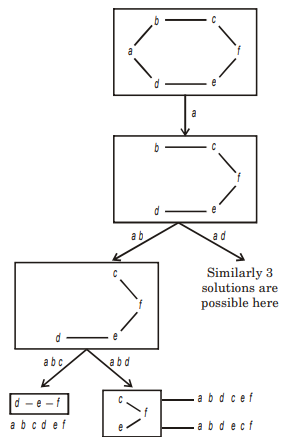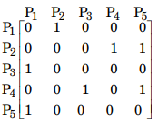Data Structures and Algorithms - Graph Algorithm

> > > > > > > > Graph Algorithm

The number of different topological ordering of the vertices of the graph is

• A

6• B

5• C

4• D

7• Option : A
• Explanation :So total solution 6

• Option : A
• Explanation :• A

4• B

7• C

23• D

99• Option : B
• Explanation :
Edge set consist edges it j = i + 1 or j = 3i
The edge sequence with minimum no of edges is
1 – 3 – 9 – 10 – 11
|
100 – 99 – 33
Which consist 7 edges

• A

Θ(n)• B

Θ(m)• C

Θ(m + n)• D

Θ(mn)• Option : C
• Explanation :
The most efficient algorithm for finding the number of connected components (articulation point) in an undirected graph on n vertices and n edges using depth-first search takes O(m + n) time.Assume n ≤ m

• A

Θ(n2)• B

Θ(n + m)• C

Θ(m2)• D

Θ(n4)• Option : B
• Explanation :
Usinng BFS traversal we can set the twin pointer in each entry in each adjacency list. So it will take O(m + n) time.

Description

• Graph Algorithms Questions can be used to give quizzes by any candidate who is preparing for UGC NET Computer Science
• This Graph Algorithms Questions section will help you test your analytical skills in a tricky method, thereby giving you an edge over other students
• Any student who wants to prepare for DOEACC A Level, DOEACC B Level, and DOEACC C level can also use these Objective Type Questions Answer.
• All candidates who have to appear for the Kendriya Vidyalaya Entrance exam can also refer to this mcq section.
• You can also get access to the Graph Algorithms MCQ ebook.
• Graph Algorithms Questions can be used in the preparation of JRF, CSIR, and various other exams.